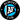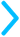## Absolute Value Equations

Absolute value equations are just like any other equation, with a slight twist. The equation splits into two versions that are like twins - almost identical, but slightly different.Check out our example below or try out our calculator.

$|x+4| = -2x + 7$
1. Split the equation: Remember that the absolute value bars make whatever is inside it positive. So, if we drop the bars, the inside of the absolute value bars can either be the positive or negative version of whatever is inside. So we have:
2. $x + 4 = -2x + 7$

#### or

$-(x + 4) = -2x + 7$
3. Solve the first equation: Without the absolute value bars, we can just solve the first equation normally:
4. \begin{aligned} x + 4 &= -2x + 7 \\ 3x &= 3 \\ x &=1 \end{aligned}
5. Solve the second equation: Next, we can solve the second equation normally:
6. \begin{aligned} -(x + 4) &= -2x + 7 \\ -x -4 &= -2x+7 \\ x &=11 \end{aligned}
7. Check your solutions: Now, we have two possible answers: $x=1$ and $x=11$.

But remember, the absolute value of something MUST be POSITIVE. So, if either of these answers result in the right side of our original equation being negative, they don't work.

Plugging in we find that if $x=1$,\begin{aligned} \mid x + 4\mid &= -2(1) +7\\ &= 5 \end{aligned}The right side is positive, so we're good. $x=1$ is a solution.

But, plugging in $x=11$,\begin{aligned} \mid x + 4\mid &= -2(11) +7\\ &= -15 \end{aligned}The right side is negative, so this can't work. $x=11$ is NOT a solution. This means our answer is just $x=1$.

And, if we graph both sides of the equation we had, we see that the black line ($|x+4|$) and the blue line ($-2x + 7$) only intersect once at $x = 1$.

### Absolute Value Calculator

Just enter in the absolute value part of the equation on the left and the rest on the right. You may need to rearrange your equation to do so.

$=$